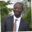Related Tags

sql
communitycreator

# How to use numeric and character data types in SQLAbel Lifaefi Mbula

## Overview

SQL (Structured Query Language) is the de facto standard language to manipulate relational database management systems. It is a strongly typed language. We must define the data types for each attribute to create the relations (tables).

This shot will discuss what a data type is and the most used data types defined by ANSI/ISO, the standardization organizations.

First, let's discuss what a data type is. A data type determines the sort of data a column can store. It helps maintain data integrity and defines allowed operations on the stored data.

Next is the data type itself. SQL supports many data types that fall into these categories:

• Numeric: integer, decimal
• Character: char, varchar
• Date and time: date, time
• Others

We primarily work with integer, decimal, float, char, and varchar in practice. Let’s learn about them in more detail.

### Numeric types

This category includes integers and floating-point numbers. There are many data types here, but we’ll focus on integer, decimal, and float.

1. Integer (or int)

int is used when we want our field (column) to store or maintain data of size 4 bytes, from -2147483648 to +2147483647. Example: Given a STUDENT table, the column that will store the age must be defined as an integer.

1. Decimal (or dec)

Dec is used to represent real numbers (with a decimal point). The syntax is as follows:

DECIMAL(M,D) or DESC(M,D)
Decimal syntax

Where (M,D) means that values can be stored with up to M digits (the precision) in total, of which D (the scale, optional) digits may be after the decimal point (or the fraction part). For example, the fees' field in the STUDENT table is to be defined in this data type.

1. Float

We use float for floating-point numbers in scientific notation. The syntax is:

FLOAT(M)
Float syntax

Where (M) specifies the minimum precision of the data.

Float is an approximate numeric data type with 4 or 8 bytes in size. The possible values range from 1.79E+308 to -2.23E-308, 0 and 2.23E-308 to 1.79E+308.

We can notice that FLOAT and DECIMAL behave almost the same. So, how do we decide to choose one rather than another? We use DECIMAL as a fixed precision numeric data type where as FLOAT is an approximate precision data type. This means that in situations where the precision is important, like in financial applications, DECIMAL must be used, and in situations where the precision is not important, we prefer FLOAT.

### Example

Let's create the STUDENT table and try to work with it as represented below:

STUDENT table with three numeric data type attributes

In the widget below, we create the table and use the DESC command to obtain info about it. Hit the Run button to see the output.

CREATE TABLE student (
age INTEGER,
fee FLOAT
);

DESC student;
Practical example for numeric types

Note: If you don't specify the argument for int, the default value is 11. For decimal, it's (10,0), 10 digits in total with no fraction part.

### Character string types

In this category, we will discuss two types, char, and varchar. Both of them can hold characters. char can hold up to 255 characters and varchar up to 65,535.

For example, in the STUDENT relation, the field NAME can be CHAR(30) or VARCHAR(30). So, what is the difference? VARCHAR is a varying character type, meaning that if we insert a name of ten characters, it will only take ten characters of space. But CHAR always takes the specified $n$ characters. In this example, a name with ten characters will occupy thirty characters of space.

#### Example

Let's add more fields to our table.

STUDENT table with six numeric data type attributes

We can implement this in the widget below.

CREATE TABLE student (
student_no INTEGER,
fullname VARCHAR(30),
age INTEGER,
country_ISO CHAR(5),
fee FLOAT
);

DESC student;
Practical example for character string types

### Summary

To sum up, we have discussed what a data type is and what are some commonly used data types. The data type specifies the type of data a column can store, an integer or a character. The data type determines the rules applied in a given column. The integer is used for whole numbers and decimal and float for a number with a decimal point. Float is less precise than decimal. Char and varchar are character string types that differ mostly in the default size occupied by the characters.

RELATED TAGS

sql
communitycreator

CONTRIBUTORAbel Lifaefi Mbula
RELATED COURSES

View all Courses

Keep Exploring

Learn in-demand tech skills in half the time